Fingerings Home Octave 1: F  (C) Gb  (Db) G  (D) Ab  (Eb) A  (E) Bb  (F) B  (Gb) C  (G) Db  (Ab) D  (A) Eb  (Bb) E  (B) Octave 2+: F  (C) Gb  (Db) G  (D) Ab  (Eb) A  (E) Bb  (F) B  (Gb) C  (G) Db  (Ab) D  (A) Eb  (Bb) E  (B) F  (C) Gb  (Db) G  (D)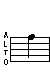F (C) - 2nd Octave## Trills

Trill - From G   Trill - From Gb
(See below for alternate)
G F   Gb F (alt)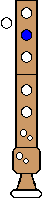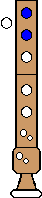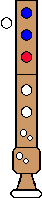Alternate Trill - From Gb
Gb (alt) F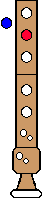Top

## Turned Trills

Turned Trill
Full step above, Full step below
G F Eb (alt) F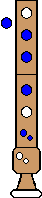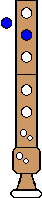Top

Turned Trill
Half step above; Full step below
Gb (alt) F Eb (alt) FTop

Turned Trill
Full step above, Half step below
G F E (alt) FComments: You can also play a normal E instead of the alternate
Top

Turned Trill
Half step above; Half step below
Gb (alt) F E (alt) FComments: You can also play a normal E instead of the alternate
Top

## Regular Turns

Turn
Full step above; Full step below
G F Eb (alt) FTop

Turn
Half step above; Full step below
Gb (alt) F Eb (alt) FComments: You can also start on a normal Gb
Top

Turn
Full step above; Half step below
G F E (alt) FComments: You can also play a normal E instead of the alternate
Top

Turn
Half step above; Half step below
Gb (alt) F E (alt) F